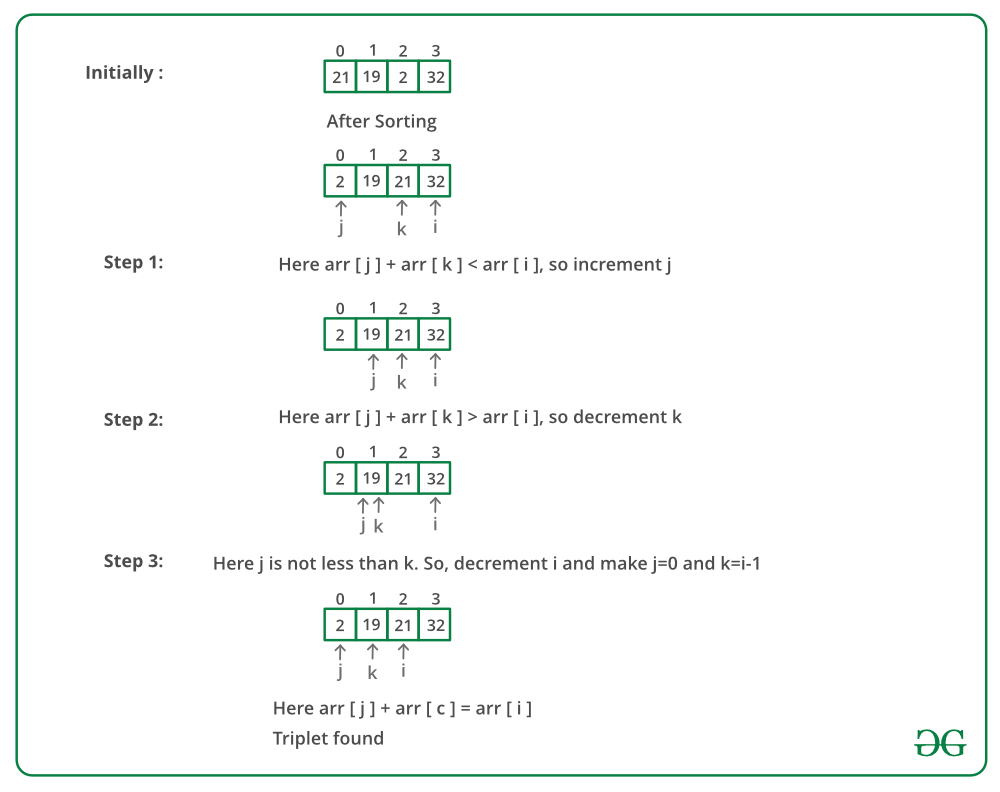# Php Program to Find a triplet such that sum of two equals to third element

• Last Updated : 11 Jan, 2022

Given an array of integers, you have to find three numbers such that the sum of two elements equals the third element.
Examples:

```Input: {5, 32, 1, 7, 10, 50, 19, 21, 2}
Output: 21, 2, 19

Input: {5, 32, 1, 7, 10, 50, 19, 21, 0}
Output: no such triplet exist```

Question source: Arcesium Interview Experience | Set 7 (On campus for Internship)

Simple approach: Run three loops and check if there exists a triplet such that sum of two elements equals the third element.
Time complexity: O(n^3)
Efficient approach: The idea is similar to Find a triplet that sum to a given value.

• Sort the given array first.
• Start fixing the greatest element of three from the back and traverse the array to find the other two numbers which sum up to the third element.
• Take two pointers j(from front) and k(initially i-1) to find the smallest of the two number and from i-1 to find the largest of the two remaining numbers
• If the addition of both the numbers is still less than A[i], then we need to increase the value of the summation of two numbers, thereby increasing the j pointer, so as to increase the value of A[j] + A[k].
• If the addition of both the numbers is more than A[i], then we need to decrease the value of the summation of two numbers, thereby decrease the k pointer so as to decrease the overall value of A[j] + A[k].

Below image is a dry run of the above approach:Below is the implementation of the above approach:

## PHP

 `= 0; ``\$i``--)``    ``{``        ``\$j` `= 0;``        ``\$k` `= ``\$i` `- 1;``        ``while` `(``\$j` `< ``\$k``) ``        ``{``            ``if` `(``\$arr``[``\$i``] == ``\$arr``[``\$j``] + ``\$arr``[``\$k``]) ``            ``{                ``                ``// Pair found``                ``echo` `"numbers are "``, ``\$arr``[``\$i``], ``" "``, ``                                      ``\$arr``[``\$j``], ``" "``, ``                                      ``\$arr``[``\$k``];``                ``return``;``            ``} ``            ``else` `if` `(``\$arr``[``\$i``] > ``\$arr``[``\$j``] + ``                                ``\$arr``[``\$k``])``                ``\$j` `+= 1;``            ``else``                ``\$k` `-= 1;``        ``}``    ``}`` ` `    ``// No such triplet is found in array``    ``echo` `"No such triplet exists"``;``}`` ` `// Driver Code``\$arr` `= ``array``(5, 32, 1, 7, 10, ``             ``50, 19, 21, 2 );``\$n` `= ``count``(``\$arr``);``findTriplet(``\$arr``, ``\$n``);``// This code is contributed by anuj_67.``?>`

Output:

`numbers are 21 2 19`

Time complexity: O(N^2)
Please refer complete article on Find a triplet such that sum of two equals to third element for more details!

My Personal Notes arrow_drop_up